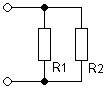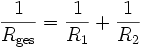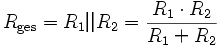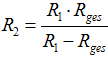# What are resistances in parallelR.totalFormula:  R.total = R1 · R2 / (R1 + R2)

You're welcome two Enter resistance values, the third value of the parallel connection will be calculated.
It can also be the total resistance R.total and a resistance R.1 or R.2 can be entered.
When entering a decimal, the is always Point to use.

Equation or formula for calculating the parallel connection of two resistors R.1 other R.2:Calculation of the necessary parallel resistance R.2, if R.1 and the total resistance R.total given is:Solving the formula R.total= (R.1· R.2) / (R.1 + R.2) to R.1:1/R.total = 1/R.1 + 1/R.2R.total·R.1·R.2[1/R.total = 1/R.1 + 1/R.2]R.1·R.2 = R.total·R.2 + R.total·R.1R.1·R.2 − R.total·R.1 = R.total · R.2R.1(R.2 − R.total) = R.2·R.totalLast step:R.1 = R.2 · R.total / (R.2 − R.total)respectively:R.2 = R.1 · R.total / (R.1 − R.total)

Note: Calculating the parallel resistances is exactly the same as calculating
the parallel connection of coils or the series connection of capacitors.• Find the resistances R.1 and R.2if the total resistance is known: •

Calculation: resistor parallel connection by iteration
Find of R.1 and R.2 if the total resistance is known● Calculate many parallel resistances ●

 Here you can calculate the total resistance - up to 10 resistors connected in parallel. Enter the resistance values ​​in the fields below and when all values ​​are entered, then click on 'Calculation'. The result is given below. As a test you can enter the values ​​4, 6 and 12 ohms; the result should be 2 ohms. Attention: Clearing the fields by hand does not delete the saved values.When entering a decimal, the is always Point to use.

Ohm's law - Ohm's law

Two resistors connected in parallel and the calculated total resistance
Resistances from 1 to 100 ohms

 R2 R1 1 1,5 2,2 3,3 4,7 6,8 10 15 22 33 47 68 1 0,5 0,6 0,69 0,77 0,83 0,87 0,91 0,93 0,95 0,97 0,98 0,99 1,5 0,6 0,75 0,89 1,03 1,14 1,22 1,30 1,36 1,40 1,43 1,45 1,46 2,2 0,69 0,89 1,1 1,32 1,50 1,66 1,82 1,92 2,0 2,06 2,10 2,13 3,3 0,77 1,03 1,32 1,65 1,94 2,22 2,48 2,70 2,87 3,00 3,08 3,14 4,7 0,83 1,14 1,50 1,94 2,35 2,78 3,20 3,58 3,87 4,12 4,27 4,39 6,8 0,87 1,22 1,66 2,22 2,78 3,40 4,05 4,68 5,19 5,64 5,94 6,18 10 0,91 1,30 1,82 2,48 3,20 4,05 5,0 6,0 6,9 7,7 8,3 8,7 15 0,93 1,36 1,92 2,70 3,58 4,68 6,0 7,50 8,9 10,3 11,4 12,2 22 0,95 1,40 2,00 2,87 3,87 5,19 6,9 8,9 11,0 13,2 15,0 16,6 33 0,97 1,43 2,06 3,0 4,12 5,64 7,7 10,3 13,2 16,5 19,4 22,2 47 0,98 1,45 2,1 3,08 4,27 5,94 8,3 11,4 15,0 19,4 23,5 27,8 68 0,99 1,46 2,13 3,14 4,39 6,18 8,7 12,2 16,6 22,2 27,8 34,0

Note: This calculator can also solve other math problems.
Calculating parallel-connected resistors is exactly the same as
those for inductors connected in parallel or capacitors connected in series.

 Converted power in a resistor: P. = U·I., P. = U2 / R., P. = I.2 ·R..

 Note: For the resistors in series is the electricity the same for each resistance and for resistors in parallel, that is tension the same for each resistor.